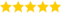# Python Operators

Last updated on 14th Oct 2020, Artciles, Blog

Shalini ((Sr Technical Project Manager ) )

Delegates in Corresponding Technical Domain with 6+ Years of Experience. Also, She is a Technology Writer for Past 3 Years & Share's this Informative Blogs for us.(5.0) | 12547 Ratings 1916

### Python Operators

Operators are used to perform operations on variables and values.

Python divides the operators in the following groups:

• Arithmetic operators
• Assignment operators
• Comparison operators
• Logical operators
• Identity operators
• Membership operators
• Bitwise operators

### 1) Arithmetic Operators

Python programming language supports different kinds of arithmetic operators for both integer and floating point like addition, subtraction, multiplication, division and so on.

Operator TypeDefinition
Subtraction (-)Subtraction operator
Multiplication (*)Multiplication operator
Division (/)Division operator
Modulus (%)Reminder operator
Floor division (//)Divides and returns the value of the remainder.
Exponentiation (**)Raises the left operand to the power of right.

### Example:

• x = 15y = 10print(‘x + y =’, x+y)

Output: x + y = 25

• print(‘x – y =’, x-y)

Output: x – y = 5

• print(‘x * y =’, x*y)

Output: x * y = 150

• print(‘x / y =’, x/y)

Output: x / y = 1.5

• print(‘x % y =’, x%y)

Output: x % y = 5

• print(‘x // y =’, x//y)

Output: x // y = 1

• print(‘x ** y =’, x**y)

Output: x ** y = 576650390625

### 2) Comparison Operators

Comparison operators are used for comparing values. It either returns True or False according to the condition.

OperatorsDefinition
Greater than (>)True if left operand is greater than the right
Less than (<)True if left operand is less than the right
Equal to (==)True if both operands are equal
Not equal to (!=)True if operands are not equal
Greater than or equal to (>=)True if left operand is greater than or equal to the right
Less than or equal to (<=)True if left operand is less than or equal to the right

### Example:

• x = 8y = 15(‘x>y is’,x>y)

Output: x > y is False

• print(‘x< y is’,  x<y)

Output: x < y is True

• print(‘x == y is’, x==y)

Output: x == y is False

• print(‘x != y is’, x!=y)

Output: x != y is True

• print(‘x >= y is’, x>=y)

Output: x >= y is False

• print(‘x<= y is’, x<=y)

Output: x <= y is True

### 3) Logical Operators

Logical operators are used for performing AND, OR and NOT operations. It either returns True or False according to the condition.

OperatorsDefinitions
andTrue if both the operands are true
orTrue if either of the operands is true
notTrue if operand is false

### Example:

• a = Trueb = Falseprint(‘a and b is’, a and b)

Output: a and b is False

• print(‘a or b is’, a or b)

Output: a or b is True

• print(‘not a is’, not a)

Output: not a is False

### 4) Bitwise Operators

Bitwise operators operate on bits and perform bit by bit operation.

OperatorsDefinitions
&Bitwise AND
|Bitwise OR
~Bitwise NOT
^Bitwise XOR
>> Bitwise right shift
<< Bitwise left shift

### 5) Assignment Operator

An assignment operator is used to assign a value to a variable.

OperatorsDefinitionsOutput
=x = 15x = 15
+=x += 15x = x + 15
-=x -= 15x = x – 15
*=x *= 15x = x * 15
/=x /= 15x = x / 15
%=x %= 15x = x % 15
//=x //= 15x = x // 15
**=x **= 15x = x ** 15
&=x &= 15x = x & 15
|=x |= 15x = x | 15
^=x ^= 15x = x ^ 15
>>=x >>= 15x = x >> 15
<<=x <<= 15x = x << 15

### 6) Identity Operators

Python offers 2 types of identity operators i.e is and is not.

Both are used to compare if two values are located on the same part of the memory. Two variables that are equal does not imply that they are identical.

OperatorsDefinitions
isTrue if the operands are identical
is notTrue if the operands are not identical

### Example:

• a1 = 3b1 = 3a2 = “Python”b2 = “Python” a3 = [4,5,6]b3 = [4,5,6] print(a1 is not b1)

Output: False

• print(a2 is b2)

Output: True

• print(a3 is b3)

Output: False

Here a3 and b3 are listed, interpreter allocates memory separately and even though they are equal, it returns False.

### 7) Membership Operators

Python offers 2 types of membership operators i.e in and not in.

Both are used to test whether a value or variable is in a sequence.

OperatorsDefinitions
inTrue if value is found in the sequence

### Example:

• a = “Python operators”b = {1:’x’,2:’y’} print(“P” in a)

Output: True

• print(“python” not in a)

Output: False

• print(1 in b)

Output: True

• print(‘y’ in b)

Output: False

1 is key and ‘x’ is the value in dictionary b. Hence, ‘y’ in b returns False.

Hope you are clear about Python operators and their various types.

Are you looking training with Right Jobs?